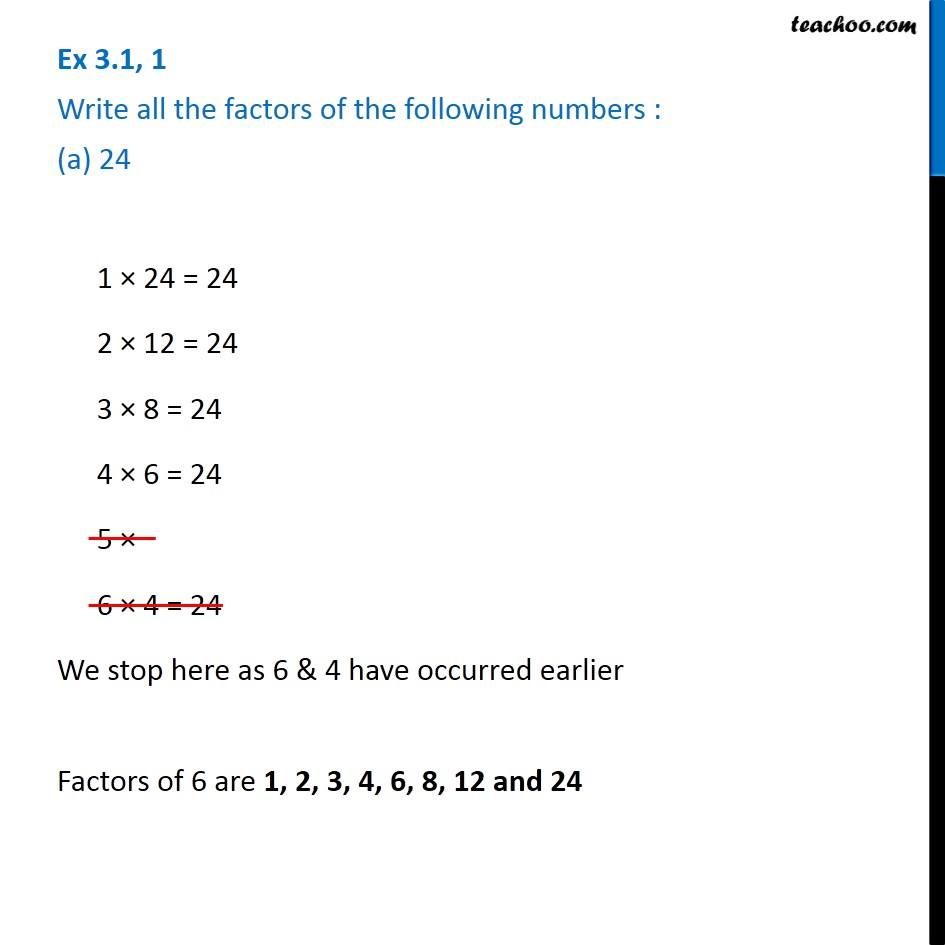1. Chapter 3 Class 6 Playing with Numbers
2. Concept wise
3. Factors and Multiples

Transcript

Ex 3.1, 1 Write all the factors of the following numbers : (a) 24 1 × 24 = 24 2 × 12 = 24 3 × 8 = 24 4 × 6 = 24 5 × 6 × 4 = 24 We stop here as 6 & 4 have occurred earlier Factors of 6 are 1, 2, 3, 4, 6, 8, 12 and 24